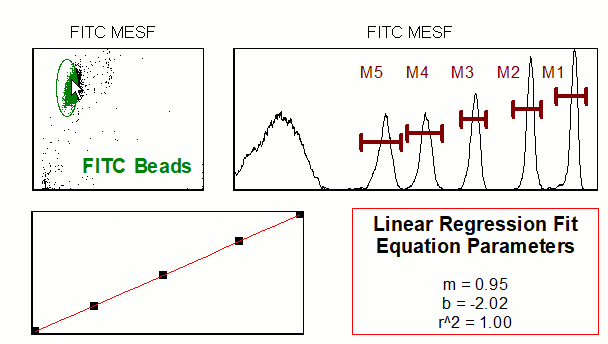## Regression Analysis

FCS Express enables the calculation of regression fits directly from your raw data, gates, and statistics. Regression formulas can easily be fit using Scatter with Regression Plots or by entering data into Spreadsheets. You can overlay a regression with the click of a button in Scatter with Regression Plots or as many multiple fit lines as you wish in a Scatter Plot.

Most important, your regression formulas, line fits, and analysis can all be linked directly to your data allowing you to view changes to your regression analysis in real-time as gates and data are adjusted. See the examples below for exploring your data in FCS Express.The linear regression above was created from a series of calibration beads.

### Explore Other Features

###### Feature Support

Have Questions? Get Help.

###### Manual and Tutorials

Regression Anlaysis in the FCS Express Manual

###### Video Resources

Regression Analysis On demand video content